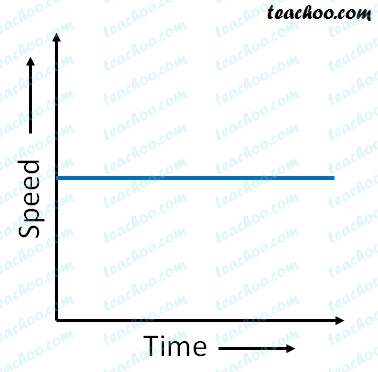Questions from Inside the chapter

Class 9
Chapter 8 Class 9 - Motion (Term 1)

## What can you say about the motion of an object if its speed time graph is a straight line parallel to the time axis?

Our graph looks likeWhen the graph of a distance-time graph is a straight line parallel to time axis,

It means that speed remains same at any time.

So, the object moves in uniform motion with constant acceleration